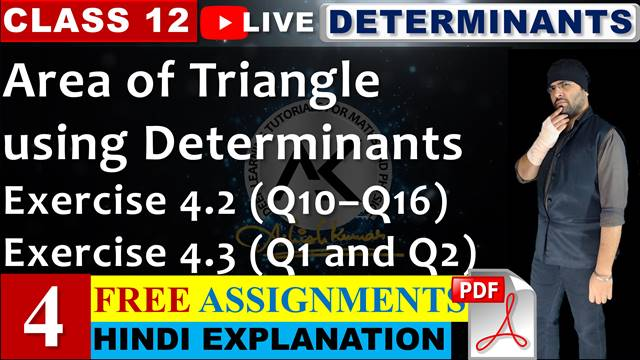# Exercise 4.2 (Q10 to Q16) Determinants Class 12 Maths Chapter 4## Topics/Questions discussed in this video:

00:00:47 NCERT Solutions Exercise 4.2 Question 10 part (i) {\begin{vmatrix} {x + 4}&{2x}&{2x}\\ {2x}&{x + 4}&{2x}\\ {2x}&{2x}&{x + 4} \end{vmatrix} = (5x + 4){{(4 - x)}^2}}

00:05:57 NCERT Solutions Exercise 4.2 Question 10 part (ii) {\begin{vmatrix} {y + k}&y&y\\ y&{y + k}&y\\ y&y&{y + k} \end{vmatrix}} = {k^2}(3y + k)

00:08:47 NCERT Solutions Exercise 4.2 Question 11 part (i) {\begin{vmatrix} {a - b - c}&{2a}&{2a}\\ {2b}&{b - c - a}&{2b}\\ {2c}&{2c}&{c - a - b} \end{vmatrix}} = {(a + b + c)^3}

00:13:27 NCERT Solutions Exercise 4.2 Question 11 part (ii) {\begin{vmatrix} {x + y + 2z}&x&y\\ z&{y + z + 2x}&y\\ z&x&{z + x + 2y} \end{vmatrix}} = 2{(x + y + z)^3}

00:18:47 NCERT Solutions Exercise 4.2 Question 12 {\begin{vmatrix} 1&x&{{x^2}}\\ {{x^2}}&1&x\\ x&{{x^2}}&1 \end{vmatrix}} = {(1 - {x^3})^2}

00:21:37 NCERT Solutions Exercise 4.2 Question 13 {\begin{vmatrix} {1 + {a^2} - {b^2}}&{2ab}&{ - 2b}\\ {2ab}&{1 - {a^2} + {b^2}}&{2a}\\ {2b}&{ - 2a}&{1 - {a^2} - {b^2}} \end{vmatrix}} = {(1 + {a^2} + {b^2})^3}

00:29:17 NCERT Solutions Exercise 4.2 Question 14 {\begin{vmatrix} {{a^2} + 1}&{ab}&{ac}\\ {ab}&{{b^2} + 1}&{bc}\\ {ca}&{cb}&{{c^2} + 1} \end{vmatrix}} = 1 + {a^2} + {b^2} + {c^2}

00:35:37 NCERT Solutions Exercise 4.2 Question 15 Let A be a square matrix of order 3 × 3, then |k\,{\rm{A}}| is equal to
(A) k| A|
(B) {k^2}|{\rm{A}}|
(C) {k^3}|{\rm{A}}|
(D) 3k|{\rm{A}}|

00:36:17 NCERT Solutions Exercise 4.2 Question 16
Which of the following is correct
(A) Determinant is a square matrix.
(B) Determinant is a number associated to a matrix.
(C) Determinant is a number associated to a square matrix.
(D) None of these

00:37:27 Area of a triangle using determinants
00:40:17 NCERT Solutions Exercise 4.3 Question 1
Find area of the triangle with vertices at the point given in each of the following:
(i) (1, 0), (6, 0), (4, 3)
(ii) (2, 7), (1, 1), (10, 8)
(iii) (–2, –3), (3, 2), (–1, –8)

00:46:22 Prove points are collinear using area of triangle and determinants

00:47:02 NCERT Solutions Exercise 4.3 Question 2
Show that points A (a, b + c), B (b, c + a), C (c, a + b) are collinear.